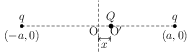# Electric Potential Energy of System of Point Charges

## Problems from IIT JEE

Problem (IIT JEE 2002): Two equal point charges are fixed at $x=-a$ and $x=+a$ on the $x$-axis. Another point charge $Q$ is placed at the origin. The change in the electrical potential energy of $Q$, when it is displaced by a small distance $x$ along the $x$-axis, is approximately proportional to,

1. $x$
2. $x^2$
3. $x^3$
4. $1/x$

Solution:Let O be the origin and $O^\prime$ be a point to the right of O at a distance $x$ (see figure). The potential at O and $O^\prime$ due to charges at $(-a,0)$ and $(a,0)$ are \begin{alignat}{2} & V_O=\frac{q}{4\pi\epsilon_0 a}+\frac{q}{4\pi\epsilon_0 a}=\frac{q}{2\pi\epsilon_0 a}, \nonumber \\ & V_{O^\prime}=\frac{q}{4\pi\epsilon_0 (a+x)}+\frac{q}{4\pi\epsilon_0 (a-x)}=\frac{q}{2\pi\epsilon_0}\left(\frac{a}{a^2-x^2}\right). \nonumber \end{alignat} The potential energy of charge $Q$ placed in a potential $V$ is $QV$. Thus, the change in potential energy of charge $Q$ when it is displaced by a small distance $x$ is, \begin{alignat}{2} \Delta U&=QV_{O^\prime}-QV_{O}=\frac{qQ}{2\pi\epsilon_0}\left[\frac{a}{a^2-x^2}-\frac{1}{a}\right]\nonumber\\ &=\frac{qQ}{2\pi\epsilon_0}\frac{x^2}{a(a^2-x^2)}\approx\frac{qQ}{2\pi\epsilon_0}\frac{x^2}{a^3}.\quad\text{(for $x\ll a$).}\nonumber \end{alignat}